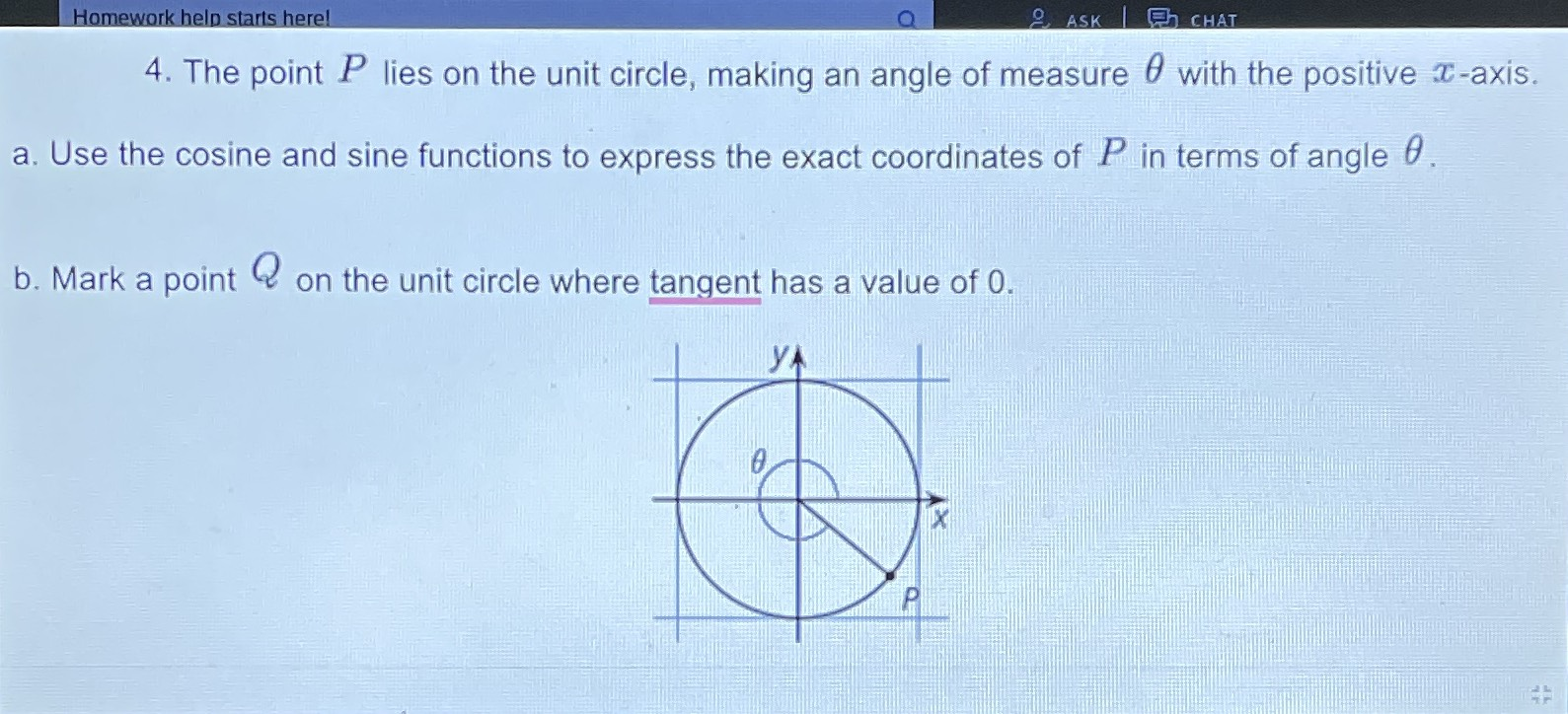### ¿Todavía tienes preguntas de matemáticas?

Pregunte a nuestros tutores expertos
Algebra
Pregunta4. The point $$P$$ lies on the unit circle, making an angle of measure $$\theta$$ with the positive $$x$$ -axis.

a. Use the cosine and sine functions to express the exact coordinates of $$P$$ in terms of angle $$\theta$$ .

b. Mark a point $$Q$$ on the unit circle where tangent has a value of $$0$$ .